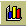### Rolling Spheres!

#### Objectives

1. Generate a best fit linear equation to data
2. Use the best fit line to make distance predictions (variable substitution and calculation)
3. Learn to solve the inverse equation to make time predictions

### Day One

#### Equipment

1. Golf or tennis ball
2. Distance measuring equipment
3. Stopwatch

#### Set Up

Measure a distance off five equal length intervals over a hard, flat, surface in a straight line.

#### Experimental Procedure

1. The group will roll a ball at a constant rate.
2. Time the ball as it passes each interval mark.
3. Record the data in the table below.

#### Table

Elapsed Time/secDistance/meters
x1: 0y1: 0
x2: ____________y2: ____________
x3: ____________y3: ____________
x4: ____________y4: ____________
x5: ____________y5: ____________

If we use the FSM China Friendship Sports Center porch, then the following tables can be used:

#### Slow run table

Elapsed Time/secDistance/meters
x1: 0y1: 0
x2: ____________y2: 7.16
x3: ____________y3: 14.33
x4: ____________y4: 21.49
x5: ____________y5: 28.65

#### Fast run table

Elapsed Time/secDistance/meters
x1: 0y1: 0
x2: ____________y2: 7.16
x3: ____________y3: 14.33
x4: ____________y4: 21.49
x5: ____________y5: 28.65

#### Homework

1. At home make an x-y graph of the data. Put the time on the horizontal x-axis, put the distance on the vertical y-axis. If we do a slow and a fast run, graph both on the same graph, using different markers for the two sets of data (mark the slow run points with an s, mark the fast run points with an f).
2. Calculate the estimated slope of the line by substituting your results into the following formula:

Slow
y5 ÷ x5

slope = _______________

Fast
y5 ÷ x5

slope = _______________

### Day Two

#### Graphing the Data using Excel

1. Launch Microsoft Excel from the Start menu.
2. Enter the data with the time in the x column and the distance in the y column from the slow run.
 A B 1 time/sec dist/meters 2 3 4 5 6
3. Use the chart wizardto make an X-Y scatter graph of the data.
4. Was the graph you did at home accurate?
5. Repeat for the fast run.

#### Calculations

1. Write down the equation provided by the slow run trendline ___________________.
2. What is the slope of the line _____________________________?
3. What is the difference between the slope you got at home and the slope from the trendline? ____________
4. Write down the equation provided by the fast run trendline ___________________.
5. What is the slope of the line _____________________________?
6. What is the difference between the slope you got at home and the slope from the trendline? ____________
7. Compare your slow and fast slopes. How does the speed of the ball relate to the slope of the line?
8. Use the fast run equation to predict the distance the ball could travel in 30 seconds.
9. Determine how long for the ball to travel 1000 meters at the fast run speed (one kilometer).

Copyright ©2003 College of Micronesia-FSM. Laboratory developed in 1997 for College and University Success Project. Revised for use in SC 130 Physical Science 07 March 2000. Altered again for use in MS 095 PreAlgebra 09 March 2003.